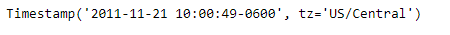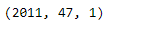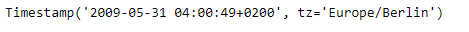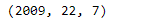# Python | Pandas Timestamp.isocalendar

• Last Updated : 14 Jan, 2019

Python is a great language for doing data analysis, primarily because of the fantastic ecosystem of data-centric python packages. Pandas is one of those packages and makes importing and analyzing data much easier.

Pandas` Timestamp.isocalendar()` function return a 3-tuple containing ISO year, week number, and weekday for the given Timestamp object.

Syntax :Timestamp.isocalendar()

Parameters : None

Return : Tuple

Example #1: Use `Timestamp.isocalendar()` function to return the date of the given Timestamp object based on ISO calendar.

 `# importing pandas as pd ` `import` `pandas as pd ` ` `  `# Create the Timestamp object ` `ts ``=` `pd.Timestamp(year ``=` `2011``,  month ``=` `11``, day ``=` `21``, ` `                  ``hour ``=` `10``, second ``=` `49``, tz ``=` `'US/Central'``) ` ` `  `# Print the Timestamp object ` `print``(ts) `

Output :Now we will use the `Timestamp.isocalendar()` function to return the date in ts object based on ISO calendar.

 `# return the date as an ISO calendar ` `ts.isocalendar() `

Output :As we can see in the output, the `Timestamp.isocalendar()` function has returned a tuple containing the year, week number and the week day for the given Timestamp object.

Example #2: Use `Timestamp.isocalendar()` function to return the date of the given Timestamp object based on ISO calendar.

 `# importing pandas as pd ` `import` `pandas as pd ` ` `  `# Create the Timestamp object ` `ts ``=` `pd.Timestamp(year ``=` `2009``, month ``=` `5``, day ``=` `31``, ` `                  ``hour ``=` `4``, second ``=` `49``, tz ``=` `'Europe/Berlin'``) ` ` `  `# Print the Timestamp object ` `print``(ts) `

Output :Now we will use the `Timestamp.isocalendar()` function to return the date in ts object based on ISO calendar

 `# return the date as an ISO calendar ` `ts.isocalendar() `

Output :As we can see in the output, the `Timestamp.isocalendar()` function has returned a tuple containing the year, week number and the week day for the given Timestamp object.

My Personal Notes arrow_drop_up
Recommended Articles
Page :